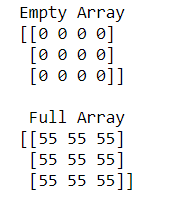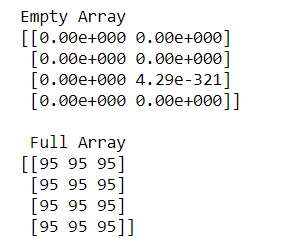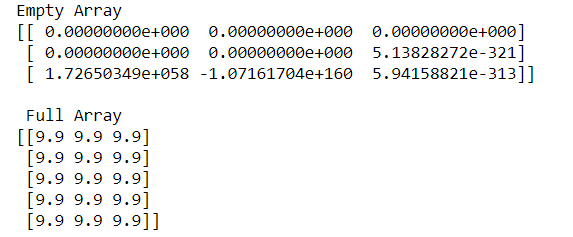Skip to content
Related Articles
How to create an empty and a full NumPy array?
• Last Updated : 01 Oct, 2020

Sometimes there is a need to create an empty and full array simultaneously for a particular question. In this situation, we have two functions named as numpy.empty() and numpy.full() to create an empty and full arrays.

Syntax:

```numpy.full(shape, fill_value, dtype = None, order = ‘C’)
numpy.empty(shape, dtype = float, order = ‘C’)
```

Example 1:

## Python3

 `# python program to create``# Empty and Full Numpy arrays`` ` `import` `numpy as np`` ` ` ` `# Create an empty array``empa ``=` `np.empty((``3``, ``4``), dtype``=``int``)``print``(``"Empty Array"``)``print``(empa)`` ` `# Create a full array``flla ``=` `np.full([``3``, ``3``], ``55``, dtype``=``int``)``print``(``"\n Full Array"``)``print``(flla)`

Output:In the above example, we create an empty array of 3X4 and full array of 3X3 of INTEGER type.

Example 2:

## Python3

 `# python program to create``# Empty and Full Numpy arrays`` ` `import` `numpy as np`` ` ` ` `# Create an empty array``empa ``=` `np.empty([``4``, ``2``])``print``(``"Empty Array"``)``print``(empa)`` ` `# Create a full array``flla ``=` `np.full([``4``, ``3``], ``95``)``print``(``"\n Full Array"``)``print``(flla)`

Output:In the above example, we create an empty array of 4X2 and full array of 4X3 of INTEGER and FLOAT type.

Example 3:

## Python3

 `# python program to create ``# Empty and Full Numpy arrays`` ` `import` `numpy as np`` ` `# Create an empty array``empa ``=`  `np.empty([``3``,``3``]) ``print``(``"Empty Array"``)``print``(empa)`` ` `# Create a full array``flla ``=` `np.full([``5``,``3``], ``9.9``)``print``(``"\n Full Array"``)``print``(flla)`

Output:In the above example, we create an empty array of 3X3 and full array of 5X3 of FLOAT type.

Attention geek! Strengthen your foundations with the Python Programming Foundation Course and learn the basics.

To begin with, your interview preparations Enhance your Data Structures concepts with the Python DS Course. And to begin with your Machine Learning Journey, join the Machine Learning – Basic Level Course

My Personal Notes arrow_drop_up